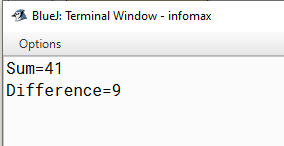# Write a program to find the sum and difference between 25 and 16 using variables in different lines

Write a program to find the sum and difference between 25 and 16 using variables in different lines.

`Solution`

``````class Demo
{
public static void main(String arr[])
{
int a=25,b=16,s,d;
s=a+b;
d=a-b;
System.out.println(“Sum=”+s);
System.out.println(“Difference=”+d);
}
}``````
`Output`